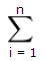Basic Circuit Problem

a) Find Va (in V) for the circuit shown on Figure#1.

Figure#1

Solution for a) :

Given i = 10 [mA] and R = 20[kΩ],

notice this circuit is a single loop circuit, therefore i = 10 [mA] everywhere on the circuit (with the given direction),

we can directly apply V = R i   ... Ohm's Law

Va = (20k)(10m)

= (20 x 103)(10 x 10-3)

= 200 [V]

Answer a) : Va = 200 [V]

Notice: Start from question b), I will use Multism (EWB) circuit design software to draw all the circuit diagrams.

b) Part1. Find equivalent resistor Req for R1 and R2 (shown on Figure #2 left)

Part2. Find equivalent resistor Req for R3 and R4 (shown on Figure #2 right)

Figure#2

Solution for b):

For part.1 Series Resistors

We can see that resistor R1 = 1[kΩ] and resistor R2 = 2 [kΩ]  are connect in "series".

For "n" resistors connect in series, the equivalent resistance are...  Req =  R1 + R2 + R3 + ... + Rn =Ri

Req = R1 + R2

= 1 + 2

= 3 [kΩ]               Answer: Req for R1 and R2 is 3 [kΩ]

For part.2 Parallel Resistors

We can see that resistor R3 = 1[kΩ] and resistor R4 = 2 [kΩ]  are connect in "parallel".

For "n" resistors connect in parallel, relationship between resistors and equivalent resistor is...     1 / Req  = 1/R1 + 1/R2 + 1/R3 + ... + 1/Rn

1 / Req = 1/ R3 + 1/ R4

=  1/1 + 1/2

=  3/2

Req     = 2 /3

= 0.67 [kΩ]

Answer: Req for R3 and R4 is approximate 0.67 [kΩ]

Now, shall we try a little harder one?

c) Find equivalent resistor Req (between point A and B) for R1, R2, R3, R4, R5 and R6 (shown on Figure #3)

Figure#3

Solution for C):

Given R1 = 1 [kΩ], R2 =2 [kΩ], R3 = 1 [kΩ], R4 = 2 [kΩ], R5 = 1 [kΩ], R6 = 5 [kΩ].

Step 1. We can combine all the same point on the circuit,

for example, look at the diagram below (Figure#3.1).

Figure#3.1

On the left hands side, we can combine point A and point B,

because there are no other sources (e.g. resistor, voltage source, diodes, ...etc) in between.

On the right hands side, we CANNOT combine point C and point D,

because there is a source in between point C and D, there we cannot combine the points.

Apply this method, we can now rearrange the circuit as below (shown on Figure#3.2)

Figure#3.2

Step 2 . Now, base on Figure#3.2 shown above.

We can see that R1 and R5, R6 and R2 are in parallel, therefore, we can find equivalent resistor for them.

1 / R1,5 = 1/R1 + 1/R5

= 1/1 + 1/1

= 2

R1,5  = 1/2

= 0.5 [kΩ]

1 / R6,2 = 1/2 + 1/5

= 5/10 + 2/10

= 7/10

R6,2 = 10/7

= 1.4286 [kΩ]

Also, we can see R3 and R4 is series connection, we can combine them too.

R3,4 = R3 + R4

= 1 + 2

= 3[kΩ]

With the information we have until now; R1,5  = 0.5[kΩ], R6,2 = 1.4286[kΩ], R3,4 = 3[kΩ].

We can re-draw the circuit as following (Shown on Figure#3.3)

Figure#3.3

Step 3. Base on Figure#3.3 shown above.

We can continue combine R1,5 and R6,2 since they connect in series.

R1,5,6,2 = R1,5 + R6,2

= 0.5 + 1.4286

= 1.9286 [kΩ]

Shown on Figure#3.4.

Figure #3.4

Last Step . Base on Figure#3.4 shown above.

We only left two resistors (R1,5,6,2 and R3,4) in parallel, combine them and find the final result.

1 / Req = 1/ R1,5,6,2 + 1/R3,4

= 1/1.9286 + 1/3

= 0.5185 + 0.3333

= 0.8518

Req = 1/0.8518

= 1.1739 [kΩ]

You may wish to use circuit design software "NI Multisim" (http://www.ni.com/multisim/ ) to do the circuit simulation.

The simulation result shown Req for circuit in Figure #3 is same as our numerical answer =1.174 [kΩ]  (Shown on Figure#3.5)

Figure #3.5

Written by Kevin Tang (Nov 2010)

[back]